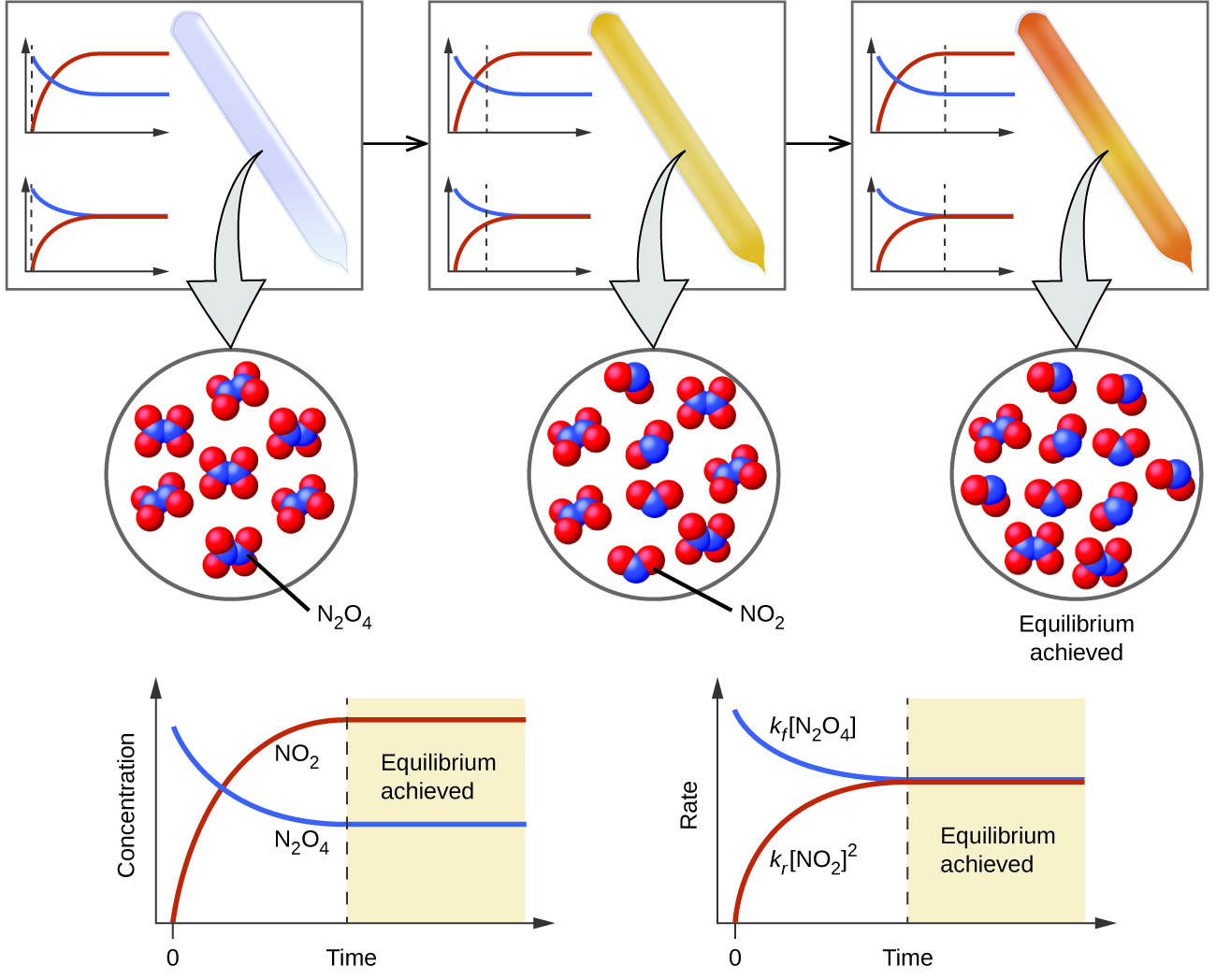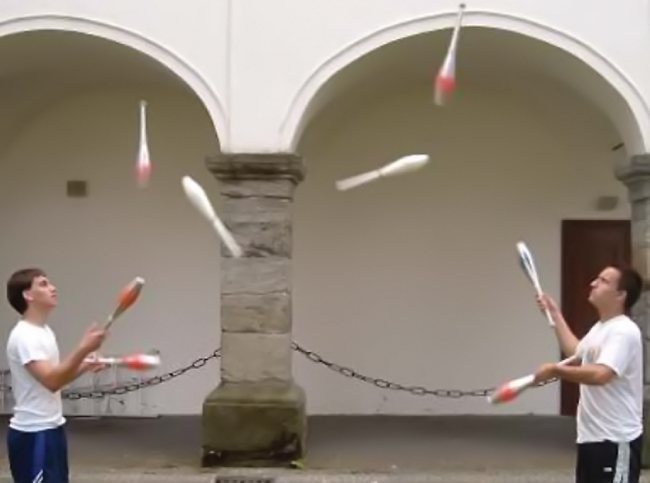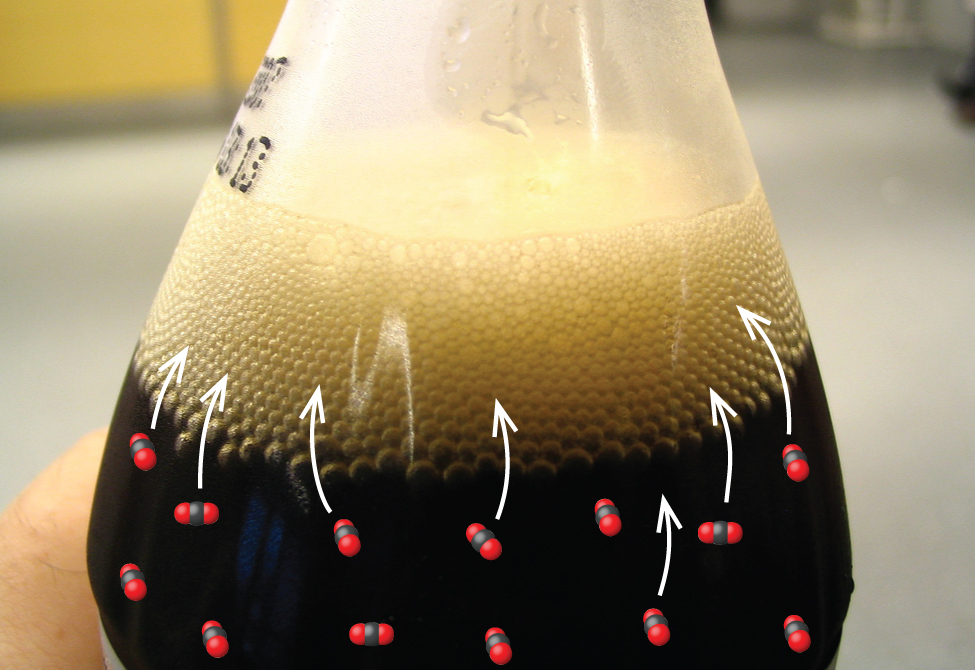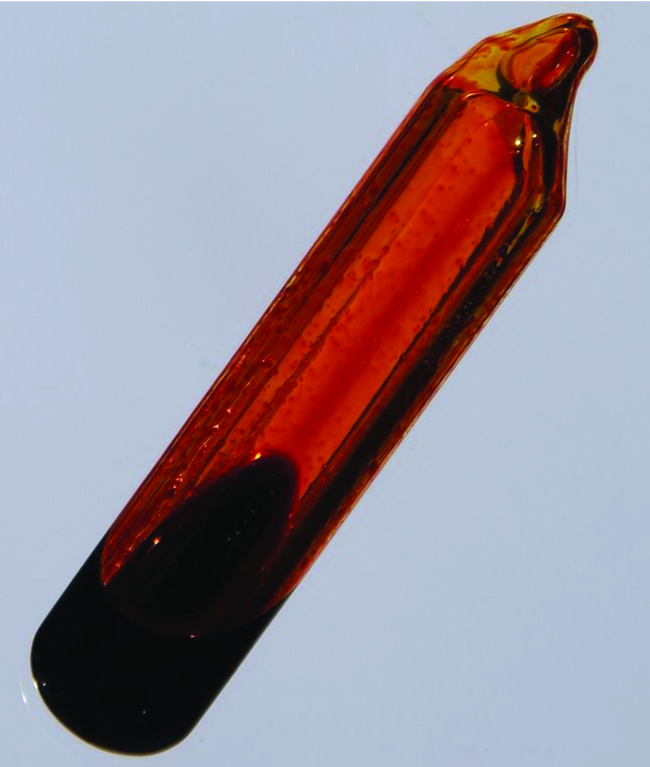Chapter 13. Fundamental Equilibrium Concepts

## 13.1 Chemical Equilibria

### Learning Objectives

By the end of this section, you will be able to:
• Describe the nature of equilibrium systems
• Explain the dynamic nature of a chemical equilibrium

A chemical reaction is usually written in a way that suggests it proceeds in one direction, the direction in which we read, but all chemical reactions are reversible, and both the forward and reverse reaction occur to one degree or another depending on conditions. In a chemical equilibrium, the forward and reverse reactions occur at equal rates, and the concentrations of products and reactants remain constant. If we run a reaction in a closed system so that the products cannot escape, we often find the reaction does not give a 100% yield of products. Instead, some reactants remain after the concentrations stop changing. At this point, when there is no further change in concentrations of reactants and products, we say the reaction is at equilibrium. A mixture of reactants and products is found at equilibrium.

For example, when we place a sample of dinitrogen tetroxide (N2O4, a colorless gas) in a glass tube, it forms nitrogen dioxide (NO2, a brown gas) by the reaction

$\text{N}_2\text{O}_4(g)\;{\rightleftharpoons}\;2\text{NO}_2(g)$

The color becomes darker as N2O4 is converted to NO2. When the system reaches equilibrium, both N2O4 and NO2 are present (Figure 1).Figure 1. A mixture of NO2 and N2O4 moves toward equilibrium. Colorless N2O4 reacts to form brown NO2. As the reaction proceeds toward equilibrium, the color of the mixture darkens due to the increasing concentration of NO2.

The formation of NO2 from N2O4 is a reversible reaction, which is identified by the equilibrium arrow (⇌). All reactions are reversible, but many reactions, for all practical purposes, proceed in one direction until the reactants are exhausted and will reverse only under certain conditions. Such reactions are often depicted with a one-way arrow from reactants to products. Many other reactions, such as the formation of NO2 from N2O4, are reversible under more easily obtainable conditions and, therefore, are named as such. In a reversible reaction, the reactants can combine to form products and the products can react to form the reactants. Thus, not only can N2O4 decompose to form NO2, but the NO2 produced can react to form N2O4. As soon as the forward reaction produces any NO2, the reverse reaction begins and NO2 starts to react to form N2O4. At equilibrium, the concentrations of N2O4 and NO2 no longer change because the rate of formation of NO2 is exactly equal to the rate of consumption of NO2, and the rate of formation of N2O4 is exactly equal to the rate of consumption of N2O4. Chemical equilibrium is a dynamic process: As with the swimmers and the sunbathers, the numbers of each remain constant, yet there is a flux back and forth between them (Figure 2).Figure 2. These jugglers provide an illustration of dynamic equilibrium. Each throws clubs to the other at the same rate at which he receives clubs from that person. Because clubs are thrown continuously in both directions, the number of clubs moving in each direction is constant, and the number of clubs each juggler has at a given time remains (roughly) constant.

In a chemical equilibrium, the forward and reverse reactions do not stop, rather they continue to occur at the same rate, leading to constant concentrations of the reactants and the products. Plots showing how the reaction rates and concentrations change with respect to time are shown in Figure 1.

We can detect a state of equilibrium because the concentrations of reactants and products do not appear to change. However, it is important that we verify that the absence of change is due to equilibrium and not to a reaction rate that is so slow that changes in concentration are difficult to detect.

We use a double arrow when writing an equation for a reversible reaction. Such a reaction may or may not be at equilibrium. For example, Figure 1 shows the reaction:

$\text{N}_2\text{O}_4(g)\;{\rightleftharpoons}\;2\text{NO}_2(g)$

When we wish to speak about one particular component of a reversible reaction, we use a single arrow. For example, in the equilibrium shown in Figure 1, the rate of the forward reaction

$2\text{NO}_2(g)\;{\longrightarrow}\;\text{N}_2\text{O}_4(g)$

is equal to the rate of the backward reaction

$\text{N}_2\text{O}_4(g)\;{\longrightarrow}\;2\text{NO}_2(g)$

### Equilibrium and Soft Drinks

The connection between chemistry and carbonated soft drinks goes back to 1767, when Joseph Priestley (1733–1804; mostly known today for his role in the discovery and identification of oxygen) discovered a method of infusing water with carbon dioxide to make carbonated water. In 1772, Priestly published a paper entitled “Impregnating Water with Fixed Air.” The paper describes dripping oil of vitriol (today we call this sulfuric acid, but what a great way to describe sulfuric acid: “oil of vitriol” literally means “liquid nastiness”) onto chalk (calcium carbonate). The resulting CO2 falls into the container of water beneath the vessel in which the initial reaction takes place; agitation helps the gaseous CO2 mix into the liquid water.

$\text{H}_2\text{SO}_4(l)\;+\;\text{CaCO}_3(s)\;{\longrightarrow}\;\text{CO}_2(g)\;+\;\text{H}_2\text{O}(l)\;+\;\text{CaSO}_4(aq)$

Carbon dioxide is slightly soluble in water. There is an equilibrium reaction that occurs as the carbon dioxide reacts with the water to form carbonic acid (H2CO3). Since carbonic acid is a weak acid, it can dissociate into protons (H+) and hydrogen carbonate ions $(\text{HCO}_3^{\;\;-})$.

$\text{CO}_2(aq)\;+\;\text{H}_2\text{O}(l)\;{\rightleftharpoons}\;\text{H}_2\text{CO}_3(aq)\;{\rightleftharpoons}\;\text{HCO}_3^{\;\;-}(aq)\;+\;\text{H}^{+}(aq)$

Today, CO2 can be pressurized into soft drinks, establishing the equilibrium shown above. Once you open the beverage container, however, a cascade of equilibrium shifts occurs. First, the CO2 gas in the air space on top of the bottle escapes, causing the equilibrium between gas-phase CO2 and dissolved or aqueous CO2 to shift, lowering the concentration of CO2 in the soft drink. Less CO2 dissolved in the liquid leads to carbonic acid decomposing to dissolved CO2 and H2O. The lowered carbonic acid concentration causes a shift of the final equilibrium. As long as the soft drink is in an open container, the CO2 bubbles up out of the beverage, releasing the gas into the air (Figure 3). With the lid off the bottle, the CO2 reactions are no longer at equilibrium and will continue until no more of the reactants remain. This results in a soft drink with a much lowered CO2 concentration, often referred to as “flat.”Figure 3. When a soft drink is opened, several equilibrium shifts occur. (credit: modification of work by “D Coetzee”/Flickr)

Let us consider the evaporation of bromine as a second example of a system at equilibrium.

$\text{Br}_2(l)\;{\rightleftharpoons}\;\text{Br}_2(g)$

An equilibrium can be established for a physical change—like this liquid to gas transition—as well as for a chemical reaction. Figure 4 shows a sample of liquid bromine at equilibrium with bromine vapor in a closed container. When we pour liquid bromine into an empty bottle in which there is no bromine vapor, some liquid evaporates, the amount of liquid decreases, and the amount of vapor increases. If we cap the bottle so no vapor escapes, the amount of liquid and vapor will eventually stop changing and an equilibrium between the liquid and the vapor will be established. If the bottle were not capped, the bromine vapor would escape and no equilibrium would be reached.Figure 4. An equilibrium is pictured between liquid bromine, Br2(l), the dark liquid, and bromine vapor, Br2(g), the orange gas. Because the container is sealed, bromine vapor cannot escape and equilibrium is maintained. (credit: http://images-of-elements.com/bromine.php)

# Key Concepts and Summary

A reaction is at equilibrium when the amounts of reactants or products no longer change. Chemical equilibrium is a dynamic process, meaning the rate of formation of products by the forward reaction is equal to the rate at which the products re-form reactants by the reverse reaction.

### Chemistry End of Chapter Exercises

1. What does it mean to describe a reaction as “reversible”?
2. When writing an equation, how is a reversible reaction distinguished from a nonreversible reaction?
3. If a reaction is reversible, when can it be said to have reached equilibrium?
4. Is a system at equilibrium if the rate constants of the forward and reverse reactions are equal?
5. If the concentrations of products and reactants are equal, is the system at equilibrium?

## Glossary

equilibrium
in chemical reactions, the state in which the conversion of reactants into products and the conversion of products back into reactants occur simultaneously at the same rate; state of balance
reversible reaction
chemical reaction that can proceed in both the forward and reverse directions under given conditions

### Solutions

Answers to Chemistry End of Chapter Exercises

1. The reaction can proceed in both the forward and reverse directions.

3. When a system has reached equilibrium, no further changes in the reactant and product concentrations occur; the reactions continue to occur, but at equivalent rates.

5. The concept of equilibrium does not imply equal concentrations, though it is possible.